# HC Verma solutions for Class 11, Class 12 Concepts of Physics Vol. 2 chapter 6 - Heat Transfer [Latest edition]

#### Chapters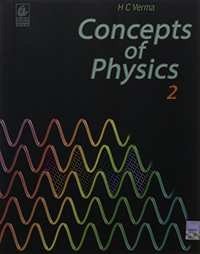## Chapter 6: Heat Transfer

Short Answers [Pages 96 - 97]

### HC Verma solutions for Class 11, Class 12 Concepts of Physics Vol. 2 Chapter 6 Heat Transfer Short Answers [Pages 96 - 97]

Short Answers | Q 1 | Page 96

The heat current is written as (ΔQ)/(Δt). Why don't we write (dQ)/dt?

Short Answers | Q 2 | Page 96

Does a body at 20°C radiate in a room, where the room temperature is 30°C? If yes, why does its temperature not fall further?

Short Answers | Q 3 | Page 96

Why does blowing over a spoonful of hot tea cools it? Does evaporation play a role? Does radiation play a role?

Short Answers | Q 4 | Page 97

On a hot summer day we want to cool our room by opening the refrigerator door and closing all the windows and doors. Will the process work?

Short Answers | Q 5 | Page 97

On a cold winter night you are asked to sit on a chair. Would you like to choose a metal chair or a wooden chair? Both are kept in the same lawn and are at the same temperature.

Short Answers | Q 6 | Page 97

Two identical metal balls one at T1 = 300 K and the other at T2 = 600 K are kept at a distance of 1 m in a vacuum. Will the temperatures equalise by radiation? Will the rate of heat gained by the colder sphere be proportional to t_2^4 - t_1^4 as may be expected from the Stefan's law?

Short Answers | Q 7 | Page 97

An ordinary electric fan does not cool the air, still it gives comfort in summer. Explain

Short Answers | Q 8 | Page 97

The temperature of the atmosphere at a high altitude is around 500°C. Yet an animal there would freeze to death and not boil. Explain.

Short Answers | Q 9 | Page 97

Standing in the sun is more pleasant on a cold winter day than standing in shade. Is the temperature of air in the sun considerably higher than that of the air in shade?

Short Answers | Q 10 | Page 97

Cloudy nights are warmer than the nights with clean sky. Explain

Short Answers | Q 11 | Page 97

Why is a white dress more comfortable than a dark dress in summer?

MCQ [Page 97]

### HC Verma solutions for Class 11, Class 12 Concepts of Physics Vol. 2 Chapter 6 Heat Transfer MCQ [Page 97]

MCQ | Q 1 | Page 97

The thermal conductivity of a rod depends on

• length

• mass

•  area of cross section

•  material of the rod

MCQ | Q 2 | Page 97

In a room containing air, heat can go from one place to another

• by conduction only

• by convection only

• by all the three modes

MCQ | Q 3 | Page 97

A solid at temperature T1 is kept in an evacuated chamber at temperature T2 > T1. The rate of increase of temperature of the body is proportional to

• T_2 - T_1

• T^2 - T^2

• T_2^3 - T_1^3

• T_2^4 - T_1^4

MCQ | Q 4 | Page 97

The thermal radiation emitted by a body is proportional to Tn where T is its absolute temperature. The value of n is exactly 4 for

•  a blackbody

• all bodies

• bodies painted black only

• polished bodies only

MCQ | Q 5 | Page 97

Two bodies A and B having equal surface areas are maintained at temperature 10°C and 20°C. The thermal radiation emitted in a given time by A and B are in the ratio

• 1 : 1.15

• 1 : 2

• 1 : 4

• 1 : 16

MCQ | Q 6 | Page 97

One end of a metal rod is kept in a furnace. In steady state, the temperature of the rod

• increases

• decreases

• remain constant

• is nonuniform

MCQ | Q 7 | Page 97

Newton's law of cooling is a special case of

• Wien's displacement law

• Kirchhoff's law

• Stefan's law

• Planck's law

MCQ | Q 8 | Page 97

A hot liquid is kept in a big room. Its temperature is plotted as a function of time. Which of the following curves may represent the plot?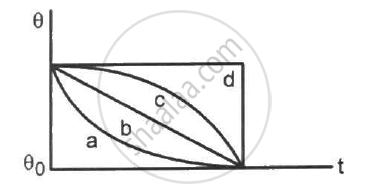• a

• b

• c

• d

MCQ | Q 9 | Page 97

A hot liquid is kept in a big room. The logarithm of the numerical value of the temperature difference between the liquid and the room is plotted against time. The plot will be very nearly

• a straight line

• a circular are

•  a parabola

• an ellipse

MCQ | Q 10 | Page 97

A body cools down from 65°C to 60°C in minutes. It will cool down from 60°C to 55°C in

•  5 minutes

• less than 5 minutes

• more than 5 minutes

•  less than or more than 5 minutes depending on whether its mass is more than or less than 1 kg.

MCQ [Pages 97 - 98]

### HC Verma solutions for Class 11, Class 12 Concepts of Physics Vol. 2 Chapter 6 Heat Transfer MCQ [Pages 97 - 98]

MCQ | Q 1 | Page 97

One end of a metal rod is dipped in boiling water and the other is dipped in melting ice.

• All parts of the rod are in thermal equilibrium with each other.

• We can assign a temperature to the rod.

• We can assign a temperature to the rod after steady state is reached

• The state of the rod does not change after steady state is reached

MCQ | Q 2 | Page 97

A blackbody does not

MCQ | Q 3 | Page 98

In summer, a mild wind is often found on the shore of a clam river. This is caused due to

• difference in thermal conductivity of water and soil.

• convection currents.

• conduction between air and the soil.

MCQ | Q 4 | Page 98

A piece of charcoal and a piece of shining steel of the same surface area are kept for a long time in an open lawn in bright sun.
(a) The steel will absorb more heat than the charcoal
(b) The temperature of the steel will be higher than that of the charcoal
(c) If both are picked up by bare hand, the steel will be felt hotter than the charcoal
(d) If the two are picked up from the lawn and kept in a cold chamber, the charcoal will lose heat at a faster rate than the steel.

MCQ | Q 5 | Page 98

A heated body emits radiation which has maximum intensity near the frequency v0. The emissivity of the material is 0.5. If the absolute temperature of the body is doubled.
(a) the maximum intensity of radiation will be near the frequency 2v0
(b) the maximum intensity of radiation will be near the frequency v0/2
(c) the total energy emitted will increase by a factor of 16
(d) the total energy emitted will increase by a factor of 8

MCQ | Q 6 | Page 98

A solid sphere and a hollow sphere of the same material and of equal radii are heated to the same temperature.
(a) Both will emit equal amount of radiation per unit time in the biginning
(b) Both will absorb equal amount of radiation from the surrounding in the biginning.
(c) The initial rate of cooling (dT/dt) will be the same for the two spheres
(d) The two spheres will have equal temperature at any instant

[Pages 98 - 102]

### HC Verma solutions for Class 11, Class 12 Concepts of Physics Vol. 2 Chapter 6 Heat Transfer [Pages 98 - 102]

Q 1 | Page 98

A uniform slab of dimension 10 cm × 10 cm × 1 cm is kept between two heat reservoirs at temperatures 10°C and 90°C. The larger surface areas touch the reservoirs. The thermal conductivity of the material is 0.80 W m−1 °C−1. Find the amount of heat flowing through the slab per minute.

Q 2 | Page 98

A liquid-nitrogen container is made of a 1 cm thick styrofoam sheet having thermal conductivity 0.025 J s−1 m−1 °C−1. Liquid nitrogen at 80 K is kept in it. A total area of 0.80 m2 is in contact with the liquid nitrogen. The atmospheric temperature us 300 K. Calculate the rate of heat flow from the atmosphere to the liquid nitrogen.

Q 3 | Page 98

The normal body-temperature of a person is 97°F. Calculate the rate at which heat is flowing out of his body through the clothes assuming the following values. Room temperature = 47°F, surface of the body under clothes = 1.6 m2, conductivity of the cloth = 0.04 J s−1 m−1°C−1, thickness of the cloth = 0.5 cm.

Q 4 | Page 98

Water is boiled in a container having a bottom of surface area 25 cm2, thickness 1.0 mm and thermal conductivity 50 W m−1°C−1. 100 g of water is converted into steam per minute in the steady state after the boiling starts. Assuming that no heat is lost to the atmosphere, calculate the temperature of the lower surface of the bottom. Latent heat of vaporisation of water = 2.26 × 106 J kg−1.

Q 5 | Page 98

One end of a steel rod (K = 46 J s−1 m−1°C−1) of length 1.0 m is kept in ice at 0°C and the other end is kept in boiling water at 100°C. The area of cross section of the rod is 0.04 cm2. Assuming no heat loss to the atmosphere, find the mass of the ice melting per second. Latent heat of fusion of ice = 3.36 × 105 J kg−1.

Q 6 | Page 98

A icebox almost completely filled with ice at 0°C is dipped into a large volume of water at 20°C. The box has walls of surface area 2400 cm2, thickness 2.0 mm and thermal conductivity 0.06 W m−1°C−1. Calculate the rate at which the ice melts in the box. Latent heat of fusion of ice = 3.4 × 105 J kg−1.

Q 7 | Page 98

A pitcher with 1-mm thick porous walls contains 10 kg of water. Water comes to its outer surface and evaporates at the rate of 0.1 g s−1. The surface area of the pitcher (one side) = 200 cm2. The room temperature = 42°C, latent heat of vaporization = 2.27 × 10J kg−1, and the thermal conductivity of the porous walls = 0.80 J s−1 m−1°C−1. Calculate the temperature of water in the pitcher when it attains a constant value.

Q 8 | Page 98

A steel frame (K = 45 W m−1°C−1) of total length 60 cm and cross sectional area 0.20 cm2, forms three sides of a square. The free ends are maintained at 20°C and 40°C. Find the rate of heat flow through a cross section of the frame.

Q 9 | Page 98

Water at 50°C is filled in a closed cylindrical vessel of height 10 cm and cross sectional area 10 cm2. The walls of the vessel are adiabatic but the flat parts are made of 1-mm thick aluminium (K = 200 J s−1 m−1°C−1). Assume that the outside temperature is 20°C. The density of water is 100 kg m−3, and the specific heat capacity of water = 4200 J k−1g °C−1. Estimate the time taken for the temperature of fall by 1.0 °C. Make any simplifying assumptions you need but specify them.

Q 10 | Page 98

The left end of a copper rod (length = 20 cm, area of cross section = 0.20 cm2) is maintained at 20°C and the right end is maintained at 80°C. Neglecting any loss of heat through radiation, find (a) the temperature at a point 11 cm from the left end and (b) the heat current through the rod. Thermal conductivity of copper = 385 W m−1°C−1.

Q 11 | Page 99

The ends of a metre stick are maintained at 100°C and 0°C. One end of a rod is maintained at 25°C. Where should its other end be touched on the metre stick so that there is no heat current in the rod in steady state?

Q 12 | Page 99

A cubical box of volume 216 cm3 is made up of 0.1 cm thick wood. The inside is heated electrically by a 100 W heater. It is found that the temperature difference between the inside and the outside surface is 5°C in steady state. Assuming that the entire electrical energy spent appears as heat, find the thermal conductivity of the material of the box.

Q 13 | Page 99

Following Figure shows water in a container having 2.0 mm thick walls made of a material of thermal conductivity 0.50 W m−1°C−1. The container is kept in a melting-ice bath at 0°C. The total surface area in contact with water is 0.05 m2. A wheel is clamped inside the water and is coupled to a block of mass M as shown in the figure. As the block goes down, the wheel rotates. It is found that after some time a steady state is reached in which the block goes down with a constant speed of 10 cm s−1 and the temperature of the water remains constant at 1.0°C. Find the mass M of the block. Assume that the heat flows out of the water only through the walls in contact. Take g = 10 m s−2.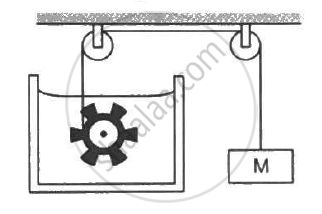Q 14 | Page 99

On a winter day when the atmospheric temperature drops to −10°C, ice forms on the surface of a lake. (a) Calculate the rate of increase of thickness of the ice when 10 cm of the ice is already formed. (b) Calculate the total time taken in forming 10 cm of ice. Assume that the temperature of the entire water reaches 0°C before the ice starts forming. Density of water = 1000 kg m−3, latent heat of fusion of ice = 3.36 × 105 J kg−1and thermal conductivity of ice = 1.7 W m−1°C−1. Neglect the expansion of water of freezing.

Q 15 | Page 99

Consider the situation of the previous problem. Assume that the temperature of the water at the bottom of the lake remains constant at 4°C as the ice forms on the surface (the heat required to maintain the temperature of the bottom layer may come from the bed of the lake). The depth of the lake is 1.0 m. Show that the thickness of the ice formed attains a steady state maximum value. Find this value. The thermal conductivity of water = 0.50 W m−1°C−1. Take other relevant data from the previous problem.

Q 16 | Page 99

Three rods of lengths 20 cm each and area of cross section 1 cm2 are joined to form a triangle ABC. The conductivities of the rods are KAB = 50 J s−1 m−1°C−1, KBC = 200 J s−1m−1°C−1 and KAC = 400 J s−1 m−1°C−1. The junctions A, B and C are maintained at 40°C, 80°C and 80°C respectively. Find the rate of heat flowing through the rods AB, AC and BC.

Q 17 | Page 99

A semicircular rod is joined at its end to a straight rod of the same material and the same cross-sectional area. The straight rod forms a diameter of the other rod. The junctions are maintained at different temperatures. Find the ratio of the heat transferred through a cross section of the semicircular rod to the heat transferred through a cross section of the straight rod in a given time.

Q 18 | Page 99

A metal rod of cross sectional area 1.0 cm2 is being heated at one end. At one time, the temperatures gradient is 5.0°C cm−1 at cross section A and is 2.5°C cm−1 at cross section B. Calculate the rate at which the temperature is increasing in the part AB of the rod. The heat capacity of the part AB = 0.40 J°C−1, thermal conductivity of the material of the rod = 200 W m−1°C−1. Neglect any loss of heat to the atmosphere

Q 19 | Page 99

Steam at 120°C is continuously passed through a 50 cm long rubber tube of inner and outer radii 1.0 cm and 1.2 cm. The room temperature is 30°C. Calculate the rate of heat flow through the walls of the tube. Thermal conductivity of rubber = 0.15 J s−1 m−1°C−1.

Q 20 | Page 99

A hole of radius r1 is made centrally in a uniform circular disc of thickness d and radius r2. The inner surface (a cylinder a length d and radius r1) is maintained at a temperature θ1 and the outer surface (a cylinder of length d and radius r2) is maintained at a temperature θ2 (θ1 > θ2). The thermal  conductivity of the material of the disc is K. Calculate the heat flowing per unit time through the disc.

Q 21 | Page 99

A hollow tube has a length l, inner radius R1 and outer radius R2. The material has a thermal conductivity K. Find the heat flowing through the walls of the tube if (a) the flat ends are maintained at temperature T1 and T2 (T2 > T1) (b) the inside of the tube is maintained at temperature T1 and the outside is maintained at T2.

Q 22 | Page 99

A composite slab is prepared by pasting two plates of thickness L1 and L2 and thermal conductivites K1 and K2. The slabs have equal cross-sectional area. Find the equivalent conductivity of the composite slab.

Q 23 | Page 99

Figure (28-E2) shows a copper rod joined to a steel rod. The rods have equal length and equal cross sectional area. The free end of the copper rod is kept at 0°C and that of the steel rod is kept at 100°C. Find the temperature at the junction of the rods. Conductivity of copper = 390 W m−1°C−1 and that if steel = 46 W m−1°C−1.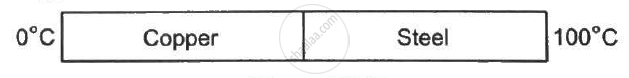Q 24 | Page 99

An aluminium rod and a copper rod of equal length 1.0 m and cross-sectional area 1 cm2 are welded together as shown in the figure . One end is kept at a temperature of 20°C and the other at 60°C. Calculate the amount of heat taken out per second from the hot end. Thermal conductivity of aluminium = 200 W m−1°C−1 and of copper = 390 W m−1°C−1.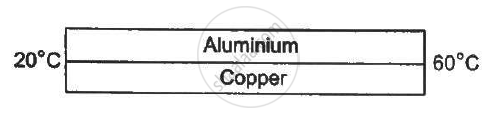Q 25 | Page 100

Following Figure shows an aluminium rod joined to a copper rod. Each of the rods has a length of 20 cm and area of cross section 0.20 cm2. The junction is maintained at a constant temperature 40°C and the two ends are maintained at 80°C. Calculate the amount of heat taken out from the cold junction in one minute after the steady state is reached. The conductivites are KAt = 200 W m−1°C−1 and KCu = 400 W m−1°C−1.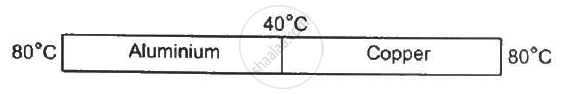Q 26 | Page 100

Consider the situation shown in the figure . The frame is made of the same material and has a uniform cross-sectional area everywhere. Calculate the amount of heat flowing per second through a cross section of the bent part if the total heat taken out per second from the end at 100°C is 130 J.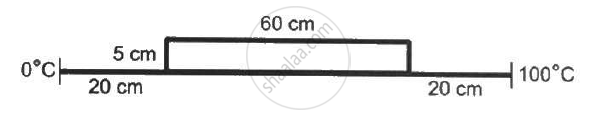Q 27 | Page 100

Suppose the bent part of the frame of the previous problem has a thermal conductivity of 780 J s−1 m−1 °C−1 whereas it is 390 J s−1 m1°C−1 for the straight part. Calculate the ratio of the rate of heat flow through the bent part to the rate of heat flow through the straight part.

Q 28 | Page 100

A room has a window fitted with a single 1.0 m × 2.0 m glass of thickness 2 mm. (a) Calculate the rate of heat flow through the closed window when the temperature inside the room is 32°C and the outside is 40°C. (b) The glass is now replaced by two glasspanes, each having a thickness of 1 mm and separated by a distance of 1 mm. Calculate the rate of heat flow under the same conditions of temperature. Thermal conductivity of window glass = 1.0 J s−1 m−1°C−1 and that of air = 0.025 m-1°C-1 .

Q 29 | Page 100

The two rods shown in following figure  have identical geometrical dimensions. They are in contact with two heat baths at temperatures 100°C and 0°C. The temperature of the junction is 70°C. Find the temperature of the junction if the rods are interchanged.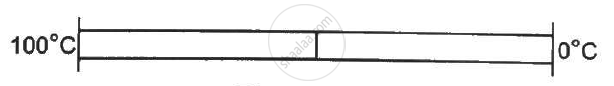Q 30 | Page 100

The three rods shown in figure  have identical geometrical dimensions. Heat flows from the hot end at a rate of 40 W in the arrangement (a). Find the rates of heat flow when the rods are joined as in arrangement (b) and in (c). Thermal condcutivities of aluminium and copper are 200 W m−1°C−1 and 400 W m−1°C−1 respectively.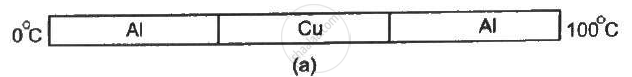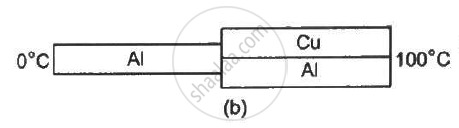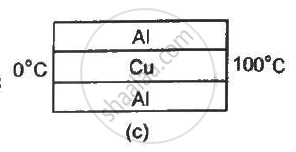Q 31 | Page 100

Four identical rods AB, CD, CF and DE are joined as shown in following figure . The length, cross-sectional area and thermal conductivity of each rod are l, A and K respectively. The ends A, E and F are maintained at temperature T1, T2 and T3 respectively. Assuming no loss of heat to the atmosphere, find the temperature at B.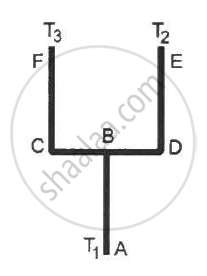Q 32 | Page 100

Seven rods A, B, C, D, E, F and G are joined as shown in the figure. All the rods have equal cross-sectional area A and length l. The thermal conductivities of the rods are KA = KC = K0, KB = KD = 2K0, KE = 3K0, KF = 4K0 and KG = 5K0. The rod E is kept at a constant temperature T1 and the rod G is kept at a constant temperature T2 (T2 > T1). (a) Show that the rod F has a uniform temperature T = (T1 + 2T2)/3. (b) Find the rate of heat flowing from the source which maintains the temperature T2.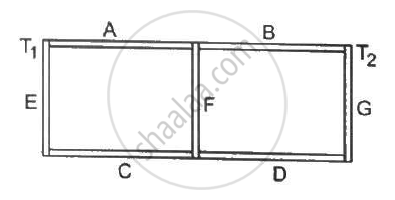Q 33 | Page 100

Find the rate of heat flow through a cross section of the rod shown in figure (28-E10) (θ2 > θ1). Thermal conductivity of the material of the rod is K.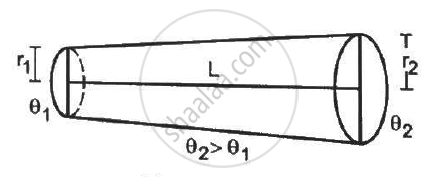Q 34 | Page 101

A rod of negligible heat capacity has length 20 cm, area of cross section 1.0 cm2 and thermal conductivity 200 W m−1°C−1. The temperature of one end is maintained at 0°C and that of the other end is slowly and linearly varied from 0°C to 60°C in 10 minutes. Assuming no loss of heat through the sides, find the total heat transmitted through the rod in these 10 minutes.

Q 35 | Page 101

A hollow metallic sphere of radius 20 cm surrounds a concentric metallic sphere of radius 5 cm. The space between the two spheres is filled with a nonmetallic material. The inner and outer spheres are maintained at 50°C and 10°C respectively and it is found that 100 J of heat passes from the inner sphere to the outer sphere per second. Find the thermal conductivity of the material between the spheres.

Q 36 | Page 101

Following figure  shows two adiabatic vessels, each containing a mass m of water at different temperatures. The ends of a metal rod of length L, area of cross section A and thermal conductivity K, are inserted in the water as shown in the figure. Find the time taken for the difference between the temperatures in the vessels to become half of the original value. The specific heat capacity of water is s. Neglect the heat capacity of the rod and the container and any loss of heat to the atmosphere.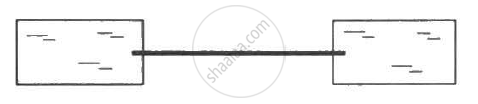Q 37 | Page 101

Two bodies of masses m1 and m2 and specific heat capacities s1 and s2 are connected by a rod of length l, cross-sectional area A, thermal conductivity K and negligible heat capacity. The whole system is thermally insulated. At time t = 0, the temperature of the first body is T1 and the temperature of the second body is T2 (T2 > T1). Find the temperature difference between the two bodies at time t.

Q 38 | Page 101

An amount n (in moles) of a monatomic gas at an initial temperature T0 is enclosed in a cylindrical vessel fitted with a light piston. The surrounding air has a temperature Ts (> T0) and the atmospheric pressure is Pα. Heat may be conducted between the surrounding and the gas through the bottom of the cylinder. The bottom has a surface area A, thickness x and thermal conductivity K. Assuming all changes to be slow, find the distance moved by the piston in time t.

Q 39 | Page 101

Assume that the total surface area of a human body is 1.6 m2 and that it radiates like an ideal radiator. Calculate the amount of energy radiated per second by the body if the body temperature is 37°C. Stefan constant σ is 6.0 × 10−8 W m−2 K−4.

Q 40 | Page 101

Calculate the amount of heat radiated per second by a body of surface area 12 cm2 kept in thermal equilibrium in a room at temperature 20°C. The emissivity of the surface = 0.80 and σ = 6.0 × 10−8 W m−2 K−4.

Q 41 | Page 101

A solid aluminium sphere and a solid copper sphere of twice the radius are heated to the same temperature and are allowed to cool under identically surrounding temperatures. Assume that the emissivity of both the spheres in the same. Find the ratio of (a) the rate of heat loss from the aluminium sphere to the rate of heat loss from the copper sphere and (b) the rate of fall of temperature of the aluminium sphere to the rate of fall of temperature of the copper sphere. The specific heat capacity of aluminium = 900 J kg−1°C−1 and that of copper = 390 J kg−1°C−1. The density of copper = 3.4 times the density of aluminium.

Q 42 | Page 101

A 100 W bulb has tungsten filament of total length 1.0 m and radius 4 × 10−5 m. The emissivity of the filament is 0.8 and σ = 6.0 × 10−8 W m−2K4. Calculate the temperature of the filament when the bulb is operating at correct wattage.

Q 43 | Page 101

A spherical ball of surface area 20 cm2 absorbs any radiation that falls on it. It is suspended in a closed box maintained at 57°C. (a) Find the amount of radiation falling on the ball per second. (b) Find the net rate of heat flow to or from the ball at an instant when its temperature is 200°C. Stefan constant = 6.0 × 10−8 W m−2 K−4.

Q 44 | Page 101

A spherical tungsten piece of radius 1.0 cm is suspended in an evacuated chamber maintained at 300 K. The piece is maintained at 1000 K by heating it electrically. Find the rate at which the electrical energy must be supplied. The emissivity of tungsten is 0.30 and the Stefan constant σ is 6.0 × 10−8 W m−2 K−4.

Q 45 | Page 101

A cubical block of mass 1.0 kg and edge 5.0 cm is heated to 227°C. It is kept in an evacuated chamber maintained at 27°C. Assuming that the block emits radiation like a blackbody, find the rate at which the temperature of the block will decrease. Specific heat capacity of the material of the block is 400 J Kg-1 K-1.

Q 46 | Page 101

A copper sphere is suspended in an evacuated chamber maintained at 300 K. The sphere is maintained at a constant temperature of 500 K by heating it electrically. A total of 210 W of electric power is needed to do it. When the surface of the copper sphere is completely blackened, 700 W is needed to maintain the same temperature of the sphere. Calculate the emissivity of copper.

Q 47 | Page 101

A spherical ball A of surface area 20 cm2 is kept at the centre of a hollow spherical shell B of area 80 cm2. The surface of A and the inner surface of B emit as blackbodies. Both A and B are at 300 K. (a) How much is the radiation energy emitted per second by the ball A? (b) How much is the radiation energy emitted per second by the inner surface of B? (c) How much of the energy emitted by the inner surface of B falls back on this surface itself?

Q 48 | Page 101

A cylindrical rod of length 50 cm and cross sectional area 1 cm2 is fitted between a large ice chamber at 0°C and an evacuated chamber maintained at 27°C as shown in the figure. Only small portions of the rod are inside the chamber and the rest is thermally insulated from the surrounding. The cross section going into the evacuated chamber is blackened so that it completely absorbs any radiation falling on it. The temperature of the blackened end is 17°C when steady state is reached. Stefan constant σ = 6 × 10−8 W m−2 K−4. Find the thermal conductivity of the material of the rod.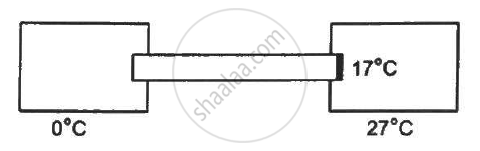Q 49 | Page 102

One end of a rod of length 20 cm is inserted in a furnace at 800 K. The sides of the rod are covered with an insulating material and the other end emits radiation like a blackbody. The temperature of this end is 750 K in the steady state. The temperature of the surrounding air is 300 K. Assuming radiation to be the only important mode of energy transfer between the surrounding and the open end of the rod, find the thermal conductivity of the rod. Stefan constant σ = 6.0 × 10−8 W m−2 K−4.

Q 50 | Page 102

A calorimeter of negligible heat capacity contains 100 cc of water at 40°C. The water cools to 35°C in 5 minutes. The water is now replaced by K-oil of equal volume at 40°C. Find the time taken for the temperature to become 35°C under similar conditions. Specific heat capacities of water and K-oil are 4200 J kg−1 K−1 and 2100 J kg−1 K−1respectively. Density of K-oil = 800 kg m−3.

Q 51 | Page 102

A body cools down from 50°C to 45°C in 5 mintues and to 40°C in another 8 minutes. Find the temperature of the surrounding.

Q 52 | Page 102

A calorimeter contains 50 g of water at 50°C. The temperature falls to 45°C in 10 minutes. When the calorimeter contains 100 g of water at 50°C, it takes 18 minutes for the temperature to become 45°C. Find the water equivalent of the calorimeter.

Q 53 | Page 102

A metal ball of mass 1 kg is heated by means of a 20 W heater in a room at 20°C. The temperature of the ball becomes steady at 50°C. (a) Find the rate of loss of heat to the surrounding when the ball is at 50°C. (b) Assuming Newton's law of cooling, calculate the rate of loss of heat to the surrounding when the ball rises 30°C. (c) Assume that the temperature of the ball rises uniformly from 20°C to 30°C in 5 minutes. Find the total loss of heat to the surrounding during this period. (d) Calculate the specific heat capacity of the metal.

Q 54 | Page 102

A metal block of heat capacity 80 J°C−1 placed in a room at 20°C is heated electrically. The heater is switched off when the temperature reaches 30°C. The temperature of the block rises at the rate of 2°C s−1 just after the heater is switched on and falls at the rte of 0.2 °C s−1 just after the heater is switched off. Assume Newton's law of cooling to hold. (a) Find the power of the heater. (b) Find the power radiated by the block just after the heater is switched off. (c) Find the power radiated by the block when the temperature of the block is 25°C. (d) Assuming that the power radiated at 25°C represents the average value in the heating process, find the time for which the heater was kept on.

Q 55 | Page 102

A hot body placed in a surrounding of temperature θ0 obeys Newton's law of cooling (d theta)/(dt) = -K(theta - theta_0)  . Its temperature at t = 0 is θ1. The specific heat capacity of the body is sand its mass is m. Find (a) the maximum heat that the body can lose and (b) the time starting from t = 0 in which it will lose 90% of this maximum heat.

## Chapter 6: Heat Transfer## HC Verma solutions for Class 11, Class 12 Concepts of Physics Vol. 2 chapter 6 - Heat Transfer

HC Verma solutions for Class 11, Class 12 Concepts of Physics Vol. 2 chapter 6 (Heat Transfer) include all questions with solution and detail explanation. This will clear students doubts about any question and improve application skills while preparing for board exams. The detailed, step-by-step solutions will help you understand the concepts better and clear your confusions, if any. Shaalaa.com has the CBSE Class 11, Class 12 Concepts of Physics Vol. 2 solutions in a manner that help students grasp basic concepts better and faster.

Further, we at Shaalaa.com provide such solutions so that students can prepare for written exams. HC Verma textbook solutions can be a core help for self-study and acts as a perfect self-help guidance for students.

Concepts covered in Class 11, Class 12 Concepts of Physics Vol. 2 chapter 6 Heat Transfer are Anomalous Expansion of Water, Heat and Temperature, Measurement of Temperature, Ideal-gas Equation and Absolute Temperature, Thermal Expansion, Calorimetry, Change of State - Latent Heat Capacity, Conduction, Convection, Radiation, Newton’s Law of Cooling, Qualitative Ideas of Blackbody Radiation, Wein'S Displacement Law, Stefan's Law, Liquids and Gases, Thermal Expansion of Solids, Green House Effect, Specific Heat Capacity.

Using HC Verma Class 12 solutions Heat Transfer exercise by students are an easy way to prepare for the exams, as they involve solutions arranged chapter-wise also page wise. The questions involved in HC Verma Solutions are important questions that can be asked in the final exam. Maximum students of CBSE Class 12 prefer HC Verma Textbook Solutions to score more in exam.

Get the free view of chapter 6 Heat Transfer Class 12 extra questions for Class 11, Class 12 Concepts of Physics Vol. 2 and can use Shaalaa.com to keep it handy for your exam preparation